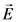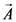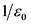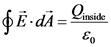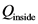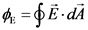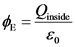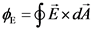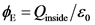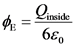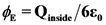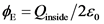# A point charge q is placed at the center of a cube of side ℓ.

General guidance

Concepts and reason

The concepts used to solve this problem are electric flux, electric field vector, charge, area vector, permittivity, and Gauss’s law.
Initially, use Gauss’s law and electric flux through a closed surface to obtain the expression for net flux of the cube.
Finally, use charge and permittivity to find the flux of the cube through each face of the cube.

Fundamentals

The expression for electric flux is given below:

Here, the flux through the closed surface is , electric field vector is , and area vector is .
According to Gauss’s law, the net electric field out of a closed surface is times the net charge enclosed by the surface.
The expression for Gauss’s law is given below:

Here, net charge is , and permittivity of free space is .

Step-by-step

Step 1 of 2

The expression for electric flux is as follows:

The expression for Gauss’s law is given below:

From the above expressions, the expression for electric flux is given below:

The net electric flux of a closed surface is equal to times the charge of the closed surface.
The flux is equal to product of electric field with the area of the surface.

The incorrect expression for electric flux is because the correct expression is .

Use the expression to find the flux through one face of the cube.

Step 2 of 2

The net flux through the surface of the cube is given below:

The point charge is in the center of the cube. Hence, each face has an equal amount of flux going through it.
The flux through one face of cube is as follows:

The flux through one face of the cube is .

The cube has six surfaces with equal area. Hence, the flux through one face is equal to the net flux divided by six.

The incorrect expression for Gauss’s law is because the correct expression is .

The flux through one face of the cube is .

The flux through one face of the cube is .

я -фв ай
ф
Е
-ӑA inside E ай- О
inside
я -фв ай
inside
я -фв ай
-ӑA inside E ай- О
inside
я – фЕхай Ёxал
я -фв ай
=Qinside/ Eo
inside
inside
= Qinside Qnide/2o
=Qinside/ Eo
We were unable to transcribe this image